# Resources tagged with: Polyhedra

Filter by: Content type:
Age range:
Challenge level:

### There are 22 results

Broad Topics > 3D Geometry, Shape and Space > Polyhedra### More Dicey Decisions

##### Age 16 to 18Challenge Level

The twelve edge totals of a standard six-sided die are distributed symmetrically. Will the same symmetry emerge with a dodecahedral die?### Tetra Inequalities

##### Age 16 to 18Challenge Level

Prove that in every tetrahedron there is a vertex such that the three edges meeting there have lengths which could be the sides of a triangle.### Euler's Formula and Topology

##### Age 16 to 18

Here is a proof of Euler's formula in the plane and on a sphere together with projects to explore cases of the formula for a polygon with holes, for the torus and other solids with holes and the. . . .### Classifying Solids Using Angle Deficiency

##### Age 11 to 16Challenge Level

Toni Beardon has chosen this article introducing a rich area for practical exploration and discovery in 3D geometry### Proximity

##### Age 14 to 16Challenge Level

We are given a regular icosahedron having three red vertices. Show that it has a vertex that has at least two red neighbours.### Three Cubes

##### Age 14 to 16Challenge Level

Can you work out the dimensions of the three cubes?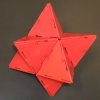### Investigating Solids with Face-transitivity

##### Age 14 to 18

In this article, we look at solids constructed using symmetries of their faces.### Sliced

##### Age 14 to 16Challenge Level

An irregular tetrahedron has two opposite sides the same length a and the line joining their midpoints is perpendicular to these two edges and is of length b. What is the volume of the tetrahedron?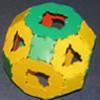### Platonic and Archimedean Solids

##### Age 7 to 16Challenge Level

In a recent workshop, students made these solids. Can you think of reasons why I might have grouped the solids in the way I have before taking the pictures?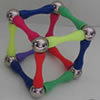### Magnetic Personality

##### Age 7 to 16Challenge Level

60 pieces and a challenge. What can you make and how many of the pieces can you use creating skeleton polyhedra?### Octa-flower

##### Age 16 to 18Challenge Level

Join some regular octahedra, face touching face and one vertex of each meeting at a point. How many octahedra can you fit around this point?### The Dodecahedron

##### Age 16 to 18Challenge Level

What are the shortest distances between the centres of opposite faces of a regular solid dodecahedron on the surface and through the middle of the dodecahedron?### Tet-trouble

##### Age 14 to 16Challenge Level

Is it possible to have a tetrahedron whose six edges have lengths 10, 20, 30, 40, 50 and 60 units?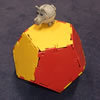### Platonic Planet

##### Age 14 to 16Challenge Level

Glarsynost lives on a planet whose shape is that of a perfect regular dodecahedron. Can you describe the shortest journey she can make to ensure that she will see every part of the planet?### The Dodecahedron Explained

##### Age 16 to 18

What is the shortest distance through the middle of a dodecahedron between the centres of two opposite faces?### Pythagoras for a Tetrahedron

##### Age 16 to 18Challenge Level

In a right-angled tetrahedron prove that the sum of the squares of the areas of the 3 faces in mutually perpendicular planes equals the square of the area of the sloping face. A generalisation. . . .### Reach for Polydron

##### Age 16 to 18Challenge Level

A tetrahedron has two identical equilateral triangles faces, of side length 1 unit. The other two faces are right angled isosceles triangles. Find the exact volume of the tetrahedron.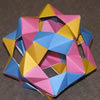### Modular Origami Polyhedra

##### Age 7 to 16Challenge Level

These models have appeared around the Centre for Mathematical Sciences. Perhaps you would like to try to make some similar models of your own.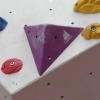### Tetra Perp

##### Age 16 to 18Challenge Level

Show that the edges AD and BC of a tetrahedron ABCD are mutually perpendicular when: AB²+CD² = AC²+BD².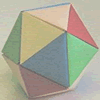### Paper Folding - Models of the Platonic Solids

##### Age 11 to 16

A description of how to make the five Platonic solids out of paper.### Dodecawhat

##### Age 14 to 16Challenge Level

Follow instructions to fold sheets of A4 paper into pentagons and assemble them to form a dodecahedron. Calculate the error in the angle of the not perfectly regular pentagons you make.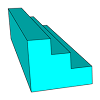### Which Solid?

##### Age 7 to 16Challenge Level

This task develops spatial reasoning skills. By framing and asking questions a member of the team has to find out what mathematical object they have chosen.